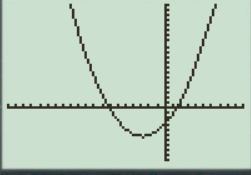# To graph : The quadratic equation y = 0.3 x 2 + 1.7 x − 3 in a graphing window.### Precalculus: Mathematics for Calcu...

6th Edition
Stewart + 5 others
Publisher: Cengage Learning
ISBN: 9780840068071### Precalculus: Mathematics for Calcu...

6th Edition
Stewart + 5 others
Publisher: Cengage Learning
ISBN: 9780840068071

#### Solutions

Chapter 1.9, Problem 14E
To determine

## To graph: The quadratic equation y=0.3x2+1.7x−3 in a graphing window.

Expert Solution

### Explanation of Solution

Given information:

Graph:

The graph of the quadratic equation y=0.3x2+1.7x3 can be sketched in the cartesian plane,

Consider the quadratic equation, y=0.3x2+1.7x3 .

Now put the values of x in the equation to find the values of y or to find the real roots of y with the help of x .

Substitute the value of x=5 in equation y=0.3+1.7x3 :

y=0.3x2+1.7x3y=0.3(5)2+1.753y=0.325+1.753y=7.58.53

The value 0f y is:

y=7.58.53y=13y=4

Substitute the value of x=4 in equation y=0.3x2+1.7x3 :

y=0.3x2+1.7x3y=0.342+1.743y=0.316+1.743y=4.86.83

The value 0f y is:

y=4.86.83y=23y=5

Substitute the value of x=2 in equation y=0.3x2+1.7x3 :

y=0.3x2+1.7x3y=0.322+1.723y=1.23.43y=5.2

Substitute the value of x=0 in equation y=0.3x2+1.7x3 :

y=0.3x2+1.7x3y=0.302+1.703y=003y=3

Observe that as the value of x increases there is slightly increment in value of y .

Steps to plot the graph of the equation y=0.3x2+1.7x3 with the help of graphing utility are as follows:

Step 1: Press MODE key.

Step 2: Use the down arrow key to reach FUNC option.

Step 3: Press ENTER key.

Step 4: Press Y= key.

Step 5: Enter the function 0.3x2+1.7x3 .

Step 6: Press WINDOW key. Change the settings to

Xmin=20Xmax=10Ymin=10Ymax=20

For better view of graph.

Step 8: Press GRAPH key.

The result obtained on the screen is provided below,Interpretation:

The equation of the function 0.3x2+1.7x3 represents a parabola.

The parabola opens upward.

The x intercepts are the points on xaxis where the graph of the equation touches xaxis .

Recall that the graphical approach to solve the equation simultaneously.

Therefore, in the equation, 0.3x2+1.7x3 there is no x intercepts.

Therefore, the equation 0.3x2+1.7x3 is symmetric about the y axis Initially the graph of the function 0.3x2+1.7x3 increases when increase the value of x ,the graph is a parabola shaped graph.

### Have a homework question?

Subscribe to bartleby learn! Ask subject matter experts 30 homework questions each month. Plus, you’ll have access to millions of step-by-step textbook answers!